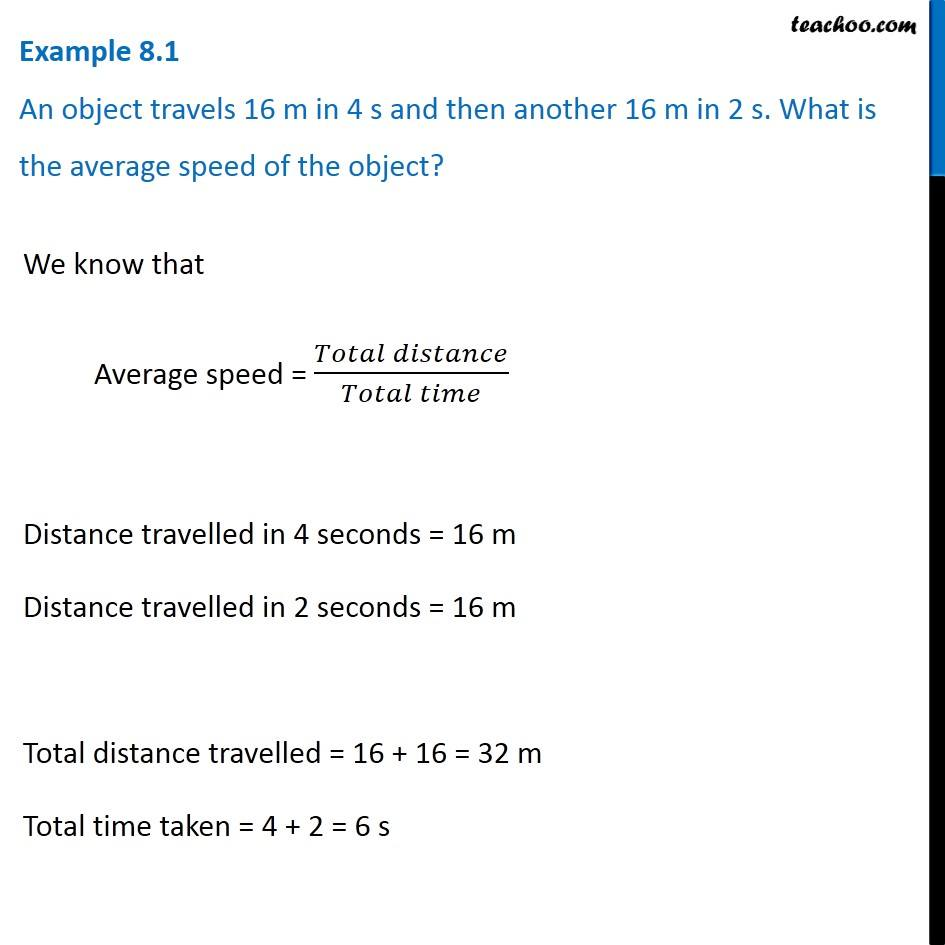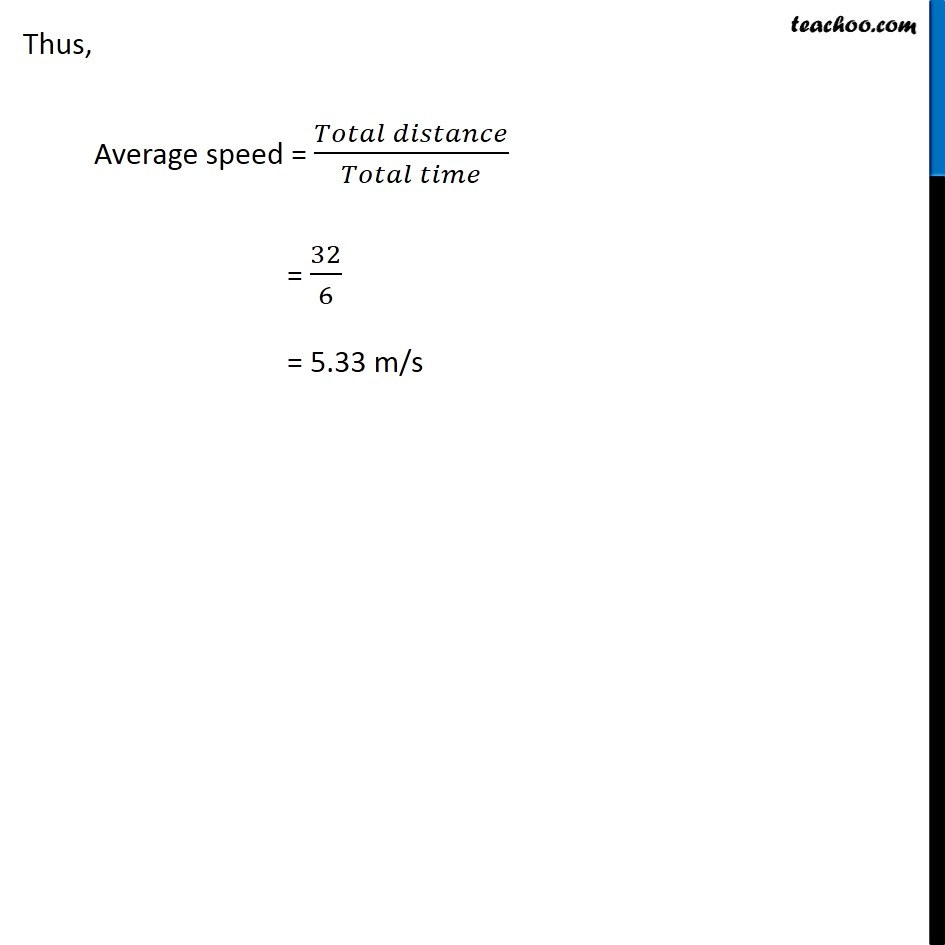Examples from NCERT Book

Class 9
Chapter 8 Class 9 - MotionLearn in your speed, with individual attention - Teachoo Maths 1-on-1 Class

### Transcript

Example 8.1 An object travels 16 m in 4 s and then another 16 m in 2 s. What is the average speed of the object? We know that Average speed = (𝑇𝑜𝑡𝑎𝑙 𝑑𝑖𝑠𝑡𝑎𝑛𝑐𝑒)/(𝑇𝑜𝑡𝑎𝑙 𝑡𝑖𝑚𝑒) Distance travelled in 4 seconds = 16 m Distance travelled in 2 seconds = 16 m Total distance travelled = 16 + 16 = 32 m Total time taken = 4 + 2 = 6 s Thus, Average speed = (𝑇𝑜𝑡𝑎𝑙 𝑑𝑖𝑠𝑡𝑎𝑛𝑐𝑒)/(𝑇𝑜𝑡𝑎𝑙 𝑡𝑖𝑚𝑒) = 32/6 = 5.33 m/s